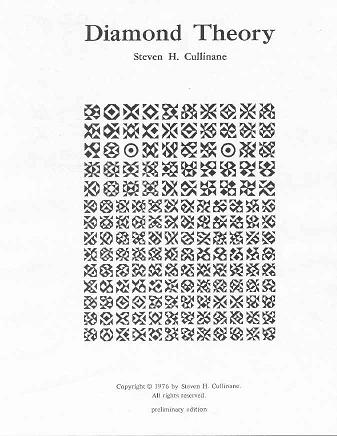Finite Geometry Notes   | Home | Site Map | Author |

 Related sites:     The 16 Puzzle    Bibliography    On the author

# Diamond Theory

#### by Steven H. Cullinane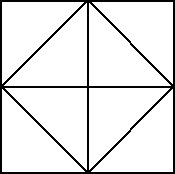Plato's Diamond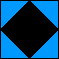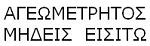Motto of

### Abstract: Symmetry in Finite Geometry

Symmetry is often described as invariance under a group of transformations. An unspoken assumption about symmetry in Euclidean 3-space is that the transformations involved are continuous.

Diamond theory rejects this assumption, and in so doing reveals that Euclidean symmetry may itself be invariant under rather interesting groups of noncontinuous (and asymmetric) transformations. (These might be called noncontinuous groups, as opposed to so-called discontinuous (or discrete) symmetry groups. See Weyl's Symmetry.)

For example, the affine group A on the 4-space over the 2-element field has a natural noncontinuous and asymmetric but symmetry-preserving action on the elements of a 4x4 array. (Details)

By embedding the 4x4 array in a 4x6 array, then embedding A in a supergroup that acts in a natural way on the larger array, one can, as R. T. Curtis discovered, construct the Mathieu group M24 -- which is, according to J. H. Conway, the "most remarkable of all finite groups."

The proof that A preserves symmetry involves the following elementary, but useful and apparently new, result: Every 4-coloring (i.e., every map into a 4-set) can be expressed as a sum of three 2-colorings. It is conceivable that this result might have applications other than to diamond theory. (Details)

The proof that A preserves symmetry also yields some insight into orthogonality of Latin squares, at least in the 4x4 case. In this case, orthogonality turns out to be equivalent to skewness of lines in a finite projective 3-space. (Details)

Diamond theory provides simple ways to visualize

• "the simplest non-trivial model for harmonic analysis,"1 the Walsh functions,
• "the smallest perfect universe,"2 the finite projective space PG(3,2),
• the "distinguished geometry," "remarkable geometric individual," and "gem,"3 the finite projective plane PG(2,4),
• the "remarkable"4 outer automorphisms of S6, and
• the structural equivalence of certain
• factorizations3 of graphs,
• parallelisms5 of partitions,
• sets of mutually orthogonal squares (i.e., n-orthogonal7 nxn matrices).

1.  F. Schipp et al., Walsh Series, 1990
2.  Burkard Polster, website
3.  A. Beutelspacher in the American Mathematical Monthly, January 1986
4.  P. J. Cameron and J. H. van Lint, Designs, Graphs, Codes, and their Links, 1991
5.  P. J. Cameron, Parallelisms of Complete Designs, 1976
6.  J. W. P. Hirschfeld, Finite Projective Spaces of Three Dimensions, 1985
7.  J. Denes and A. D. Keedwell, Latin Squares and their Applications, 1974

As a bonus, extending the action of A to a 4x4x4 array yields a simple way of generating the 1.3 trillion transformations natural to the 64 hexagrams of the I Ching. (Details)#### Mathematics Subject Classification (MSC2000) - Primary: 20B25, Finite automorphism groups of algebraic, geometric, or combinatorial structures. Secondary: 05B25, Finite geometries; 51E20, Combinatorial structures in finite projective spaces.

The following diagram is a rough sketch of how diamond theory is related to some other fields of mathematics.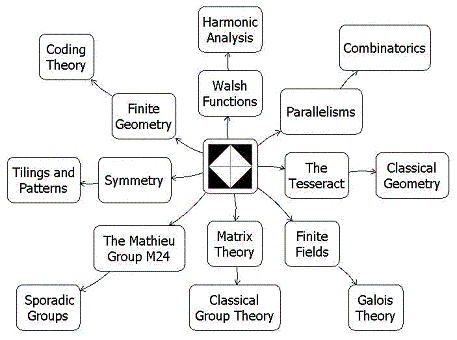### To Shirley

On Euclid's Elements:
"In view of... admiration the Elements has consistently elicited over the years, and of the prestigious role it concomitantly maintained until the mid-19th century as scientific archetype, it is not surprising that we find in the history of philosophy a concept of truth sustained by the example of the Elements, whose influence in philosophy runs parallel to that of the Elements in science. This concept I will call the 'Diamond Theory' of truth."
-- Richard J. Trudeau in The Non-Euclidean Revolution, 1987

## Introduction:

The image below shows the cover of a booklet I wrote in 1976. The booklet details the implications of what I call the "diamond theorem," after the diamond figure in Plato's Meno dialogue. This website, which updates the booklet, is written for mathematicians and college students of mathematics. For a less technical treatment of philosophical and literary matters related to the diamond theorem, see The Diamond Theory of Truth.